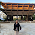Synthesizable RTC in VHDL

The code given is only for clock digit incrementation. For components in clock like min_clk and sec_clk, refer my previous codes.

VHDL code:

library ieee;
use ieee.std_logic_1164.all;
use ieee.numeric_std.all;

entity clk12 is
port(
clk: in std_logic;
rst: in std_logic;
op: out std_logic;
op0,op1,op2,op3: out std_logic_vector(6 downto 0)
);

end clk12;

architecture clock of clk12 is

component sec_clk
Port (
clk : in std_logic;
op  : out std_logic
);
end component;

component min_clk
Port (
clk : in std_logic;
rst : in std_logic;
op  : out std_logic
);
end component;

component seg7
port(m: in integer range 0 to 10;
num: out std_logic_vector(6 downto 0));
end component;

signal flag: std_logic;
signal sflag: std_logic;

signal a: integer range 0 to 10;
signal b: integer range 0 to 6;
signal c: integer range 0 to 10;
signal d: integer range 0 to 3;

begin

c1: sec_clk port map(clk,sflag);
c2: min_clk port map(sflag,rst,flag);
op<=sflag;

process(flag,rst)

variable m0: integer range 0 to 10:=0;
variable m1: integer range 0 to 6:=0;
variable m2: integer range 0 to 10:=2;
variable m3: integer range 0 to 3:=1;

begin
a<=m0;
b<=m1;
c<=m2;
d<=m3;
if rst='0' then
m0:=0;
m1:=0;
m2:=2;
m3:=1;
elsif rising_edge(flag) then
if  m0/=9 then
m0:= m0+1;
elsif  m0=9 and  m1/=5  then
m0:=0;
m1:= m1+1;
elsif  m0=9 and  m1=5 and  m2/=9 and  m3=0 then
m0:=0;
m1:=0;
m2:= m2+1;
elsif  m0=9 and  m1=5 and  m2=9 and  m3=0 then
m0:=0;
m1:=0;
m2:=0;
m3:=1;
elsif  m3=1 and  m2/=2 and  m1=5 and  m0=9 then
m2:= m2+1;
elsif  m3=1 and  m2=2 and  m1=5 and  m0=9 then
m0:=0;
m1:=0;
m2:=1;
m3:=0;
end if;
end if;

end process;

z0: seg7 port map(a,op0);
z1: seg7 port map(b,op1);
z2: seg7 port map(c,op2);
z3: seg7 port map(d,op3);

end clock;

Note: The clock cant be set manually. It starts from 12:00. I tried my best to add the manual set function but didnt succeed. But I assure that manual set will also be there in couple of time. Suggetions are most welcome.

Quartus SOF file for DE1 board only: Digiclk(for DE1 board only).SOF

Check the new code with hr and min manual set: RTC with maual set

Enjoy Programming.

1.hello. i just want to ask if by any chance, you know how to display the clock in an external LCD panel. like a 2-line 16 pin LCD panel. I'm having a hard time in displaying the desired output.

RTC on FPGA with manual set synthesizeble VHDL code.

Hey there, cheers to VHDL. I successfully added manual set feature in my previous RTC project. I am not going to copy whole code here. I am just adding the links of .vhd files. I also added the pre-compiled .sof file only for DE1 boards. There is a very small bug that while clicking the set button to enable min and hr set, it increments the hr or min value unintentionally. Else everything is working great.

(Input freq 24MHz., top-level entity DigiClock)

VHDL code for Clock Divider

This is a clock divider code, just set the max-count value as per your requirenment.

For ex. If I want 1Hz freq. set the max count to i/p freq value viz.
1sec = 1Hz
Then, to get time period of 1sec i.e. 1 Hz frequency set max-count to 240000 as shown below:

1sec  =  24000000  -- for i/p frequency of 24 MHz.

To get your desired frequency just calculate the maxcount with the formula given below:

max_count = 24000000 * (1/your required frequency)

CODE:

1 2 3 4 5 6 7 8 9 10 11 12 13 14 15 16 17 18 19 20 21 22 23 24 25 26 27 28 29 30 31 32 33 34 35 36 37 38 39 40 41 42 43 44 45 46 47 48libraryIEEE; useIEEE.STD_LOGIC_1164.ALL; useIEEE.numeric_std.all; entityclk_divisPort ( Clk :instd_logic; rst :instd_logic; op :outstd_logic ); endclk_div; architecturebehavioralofclk_divisconstant max_count :natural:=24000000; -- I used 24MHz clockbeginprocess(Clk,rst) variable count :naturalrange0to max_count; beginif rst ='0'…